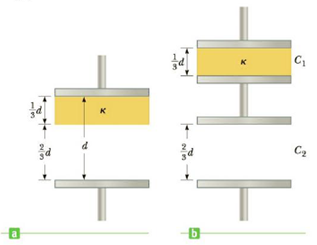Chapter 16, Problem 61AP

Chapter
Section
Textbook Problem

A parallel-plate capacitor with a plate separation d has a capacitance C0 in the absence of a dielectric. A slab of dielectric material of dielectric constant κ and thickness d/3 is then inserted between the plates as in Figure P16.61a. Show that the capacitance of this partially filled capacitor is given by C = ( 3 κ 2 κ + 1 ) C 0 Hint: Treat the system as two capacitors connected in series as in Figure P16.61b, one with dielectric in it and the other one empty.Figure P16.61

To determine
The equivalent capacitance.

Explanation

The capacitors are in series combination.

Formula to calculate the equivalent capacitance of two capacitors in series is,

1Ceq=1C1+1C2

Formula to calculate C1 is,

C1=κε0A(d/3)

Formula to calculate C2 is,

C2=ε0A(2d/3)

From the above equations,

1Ceq=d3κε0A<

Still sussing out bartleby?

Check out a sample textbook solution.

See a sample solution

The Solution to Your Study Problems

Bartleby provides explanations to thousands of textbook problems written by our experts, many with advanced degrees!

Get Started

How do eating disorders affect health?

Understanding Nutrition (MindTap Course List)

What is motion?

An Introduction to Physical Science

What are sister chromatids?

Human Heredity: Principles and Issues (MindTap Course List)